2014-04-17

Simplified DES是由Edard Schaefer教授开发的用于教学的简单加密算法，它与DES有着相似的属性与结构，只是参数比较小而已。通过这种简单的，能够实现手工加解密的算法能让我们加深对DES的理解。

### 概述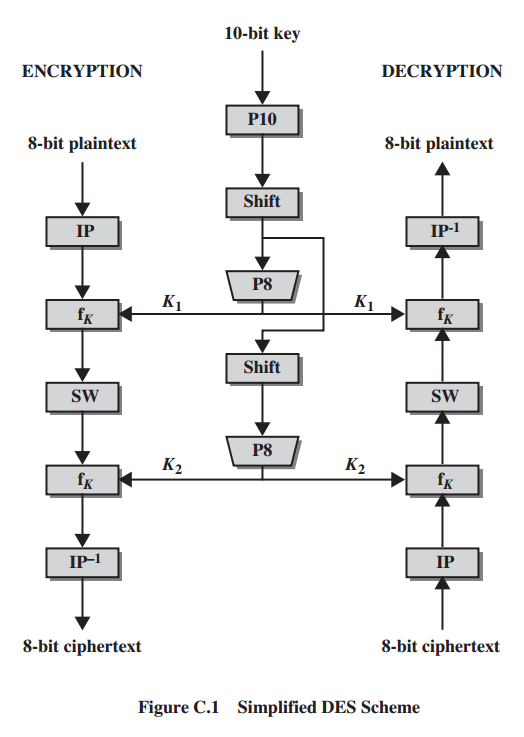$$f{}_{k}$$的输入包括明文和8位密钥，我们可以使用16位的密钥，每一轮使用8位（共两轮$$f{}_{k}$$），也可以使用8位密钥，每一次都使用相同的密钥。作为折中，我们使用了10位密钥，每一次的$$f{}_{k}$$通过移位产生8位密钥。两个密钥的产生如图2：$IP^{-1}\circ f_{K_{2}}\circ SW\circ f_{K_{1}}\circ IP$

$ciphertext = IP^{-1}(f_{K_{2}}(SW(f_{K_{1}}(IP(plaintext)))))$

$K_{1} = P8(Shift(P10(key))) K_{2} = P8(Shift(Shift(P10(key))))$

$plaintext = IP^{-1}(f_{K_{1}}(SW(f_{K_{2}}(IP(ciphertext)))))$

### S-DES密钥生成

$P10(k_{1},k_{2},k_{3},k_{4},k_{5},k_{6},k_{7},k_{8},k_{9},k_{10}) = (k_{3},k_{5},k_{2},k_{7},k_{4},k_{10},k_{1},k_{9},k_{8},k_{6})$

P10可以简单表示如下：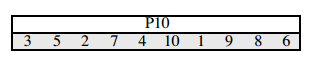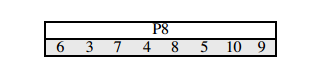### S-DES加密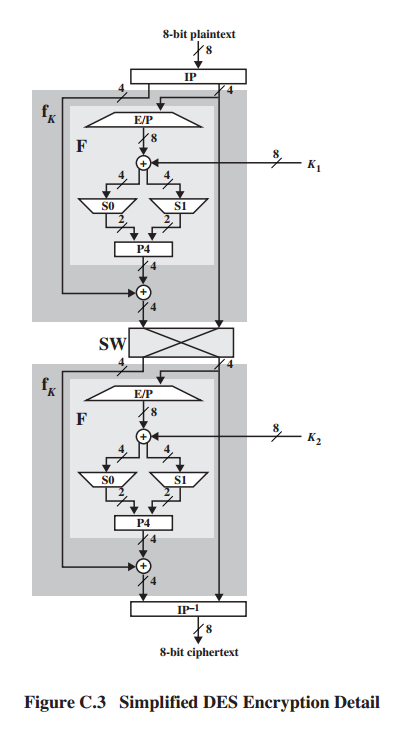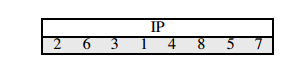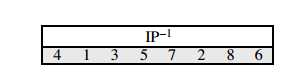$$f_{K}$$函数：

S-DES中最复杂的部分就是函数$$f_{K}$$了，这个函数包含了置换的和替换的组合。这个函数解释如下。首先让L和R分别表示$$f_{K}$$8位输入的左边4位和右边4位，F是一个4位到4位的映射。则

$f_{K}(L,R) = (L\oplus F(R,SK),R)$

SK是一个子密钥。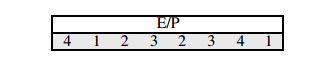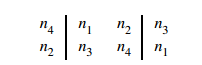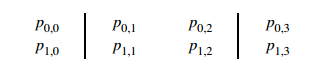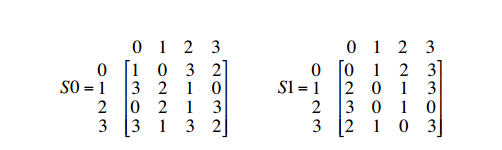S盒操作如下：第一个和第四个输入作为一个2位数指定S盒中的一行，第二和第三个输入作为一个2位数指定S盒中的一列。比如$$(p_{0,0}p_{0,3})=(00)$$ 和$$(p_{0,1}p_{0,2})=(10)$$ ，则输出是S盒的第一行第二列这里是3（二进制的11），类似的$$(p_{1,0}p_{1,3})$$ 和$$(p_{1,1}p_{1,2})$$的值找到在第二个S盒中的值。

$$P4$$的输出就是F的输出。

SW函数：

$$f_{K}$$函数仅仅处理左边的4位，SW函数将左右互换然后就处理了后面的4位。第二轮的$$f_{K}$$中，E/P,S0,S1和P4都跟第一轮一样的，只有子密钥变成了$$K_{2}$$。

S-DES